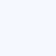Home » Technical Diving Articles » Technical Diving Theory Knowledgebase » Calculating No Stop Time for Scuba Diving

# Calculating ‘No Stop Time’

by Erik C. Baker, P.E.

The following is an explanation about calculating the “No Stop Time”:

Starting out with the basic Haldane equation (keep in mind that this equation is for a CONSTANT DEPTH profile, not for ascents or descents):

P = Po + (Pi – Po)(1 – e^-kt)

which states that P, the final partial pressure in a given compartment (as a function of time), will be the initial partial pressure, Po, plus (or minus) the exponential gas loading that takes place. The exponential gas loading is given by the second part of the equation, (Pi – Po)(1 – e^-kt).
Note that Pi – Po is the pressure gradient between the inspired gas pressure and the initial compartment pressure. This is the “driving force” for on-gassing and off-gassing.

You can rearrange the Haldane equation to solve for time, t:

(P – Po)/(Pi – Po) = 1 – e^-kt

e^-kt = 1 – (P – Po)/(Pi – Po)

before you take the natural logarithm of both sides, you should simplify the equation further (you did not do this in your post):

e^-kt = (Pi – Po)/(Pi – Po) – (P – Po)/(Pi – Po)

e^-kt = [(Pi – Po)-(P – Po)]/(Pi – Po)

e^-kt = (Pi – Po – P + Po)/(Pi – Po)

e^-kt = (Pi – P)/(Pi – Po)

now take the natural logarithm of both sides:

ln[e^-kt] = ln[(Pi – P)/(Pi – Po)]

-kt = ln[(Pi – P)/(Pi – Po)]

t = (-1/k)*ln[(Pi – P)/(Pi – Po)]

this time, t, is the time that it will take for the compartment to load gas (on-gas or off-gas) from the initial compartment pressure, Po, to the final pressure, P.

You can substitute the surfacing M-value, Mo, for the final pressure, P:

t = (-1/k)*ln[(Pi – Mo)/(Pi – Po)]

In this case, the time, t, is the “No Stop Time” or “No Decompression Limit” (NDL) based on the present gas loading, Po, and the inspired gas pressure, Pi.

Example:

A diver begins an air dive by descending to a depth of 30 meters of seawater (msw) very rapidly. Let’s assume that it was an “instantaneous” descent. Now he is on the bottom at 30 msw. What is the “No Stop Time” or “No  Decompression Limit” for this depth?

Note: Remember all pressures are ABSOLUTE!

Assuming the dive is from sea level, the ambient pressure is the depth pressure of 30 msw plus the surface barometric pressure of 10 msw:

Pamb = 30 msw + 10 msw = 40 msw

The inspired pressure is the ambient pressure minus the water vapor pressure (Buhlmann value) times the fraction of inert gas:

Pi = (Pamb – PH2O)*FN2 = (40 msw – 0.627 msw)*0.79 = 31.1 msw

The initial compartment pressure will be due to breathing atmospheric air at the surface for a long time (first dive of the day):

Po = (Pamb – PH20)*FN2 = (10 msw – 0.627 msw)*0.79 = 7.4 msw

The surfacing M-values will vary by compartment. See Table 2 in my “Understanding M-values” article. Example: for Compartment No. 2 using the Buhlmann ZH-L16B M-values, Mo = 25.4 msw.

The time constant, k, for Compartment No. 2 is the natural logarithm of 2 divided by the half-time:

k (Cpt 2) = ln2/half-time = 0.693/8 min = 0.086625

Now we can compute the “No Stop Time” or NDL for this compartment:

t = (-1/k)*ln[(Pi – Mo)/(Pi – Po)]

t (Cpt 2) = (-1.0/0.086625)*ln[(31.1 – 25.4)/(31.1 – 7.4)]

t (Cpt 2) = 16.45 minutes

This process is repeated for each compartment and the shortest time computed across all compartments is the NDL for the profile.

VERY IMPORTANT NOTE: You cannot apply the “No Stop Time” equation,

t = (-1/k)*ln[(Pi – Mo)/(Pi – Po)], under the following conditions:

1. If the quantity within the logarithm bracket is a negative number. This is because the logarithm of a negative number is a complex number (i.e. a + ib) which is NOT A REAL situation for diving!

The quantity within the logarithm bracket will be negative if:

a. Mo > Pi > Po
b. Mo < Pi < Po

2. If Pi = Po then there will be division by zero which cannot be accomplished.

3. If the quantity within the logarithm bracket is a positive number greater than one (1), then the time computed in the overall equation will be negative, which is NOT A REAL situation for diving!

The quantity within the logarithm bracket will be positive and greater than one (1) if:

c. Mo < Pi > Po
d. Mo > Pi < Po

THEREFORE, the “No Stop Time” equation, t = (-1/k)*ln[(Pi – Mo)/(Pi – Po)],
can only be applied when,

A. Pi > Mo > Po (on-gassing) or
B. Pi < Mo < Po (off-gassing)

In other words, Mo, has to be a value between the present value of Pi and Po.

For the typical sport diving application, the “No Stop Time” equation will be applied only for condition A above, that is when,

A. Pi > Mo > Po (on-gassing)

The program must include the logic to account for this requirement. An example
of this is as follows:

NOSTOP = 0.0
DO 100 I = 1,16
IF ((PIN2 .GE. MVALUE(I)) .AND. (MVALUE(I) .GT. PN2O(I)) THEN TIME(I) = -1.0/KN2(I)*ALOG((PIN2 – MVALUE(I))/(PIN2 – PN2O(I)))
NOSTOP = MIN(NOSTOP, TIME(I))
END IF
100 CONTINUE

The “No Stop Time” calculation will be applied after each change in depth during the dive (if it is being calculated in real-time). Of course, the dive computer will still need to keep track of the gas loadings separately during the dive as well.

Originally posted 2018-03-07 23:54:23.